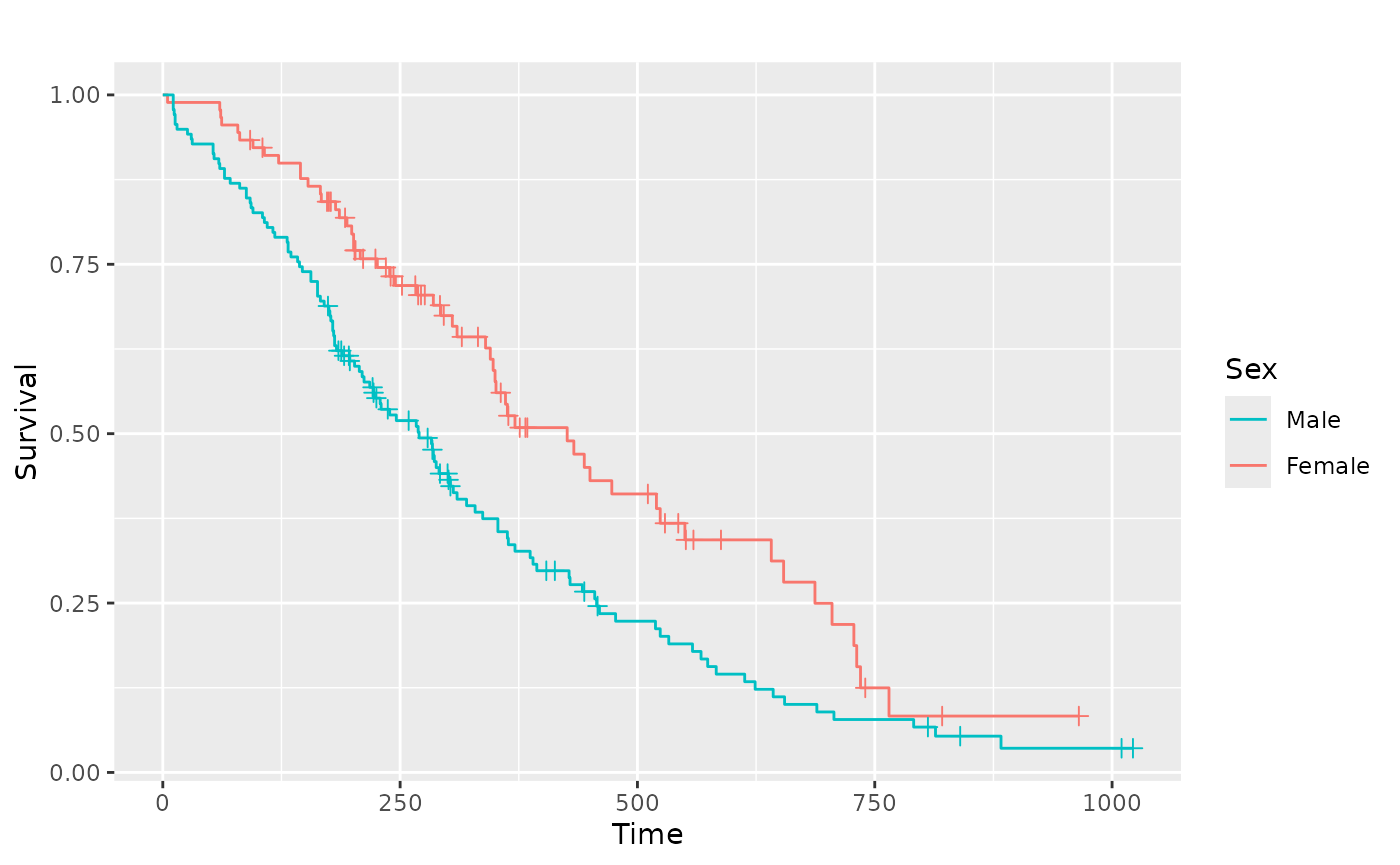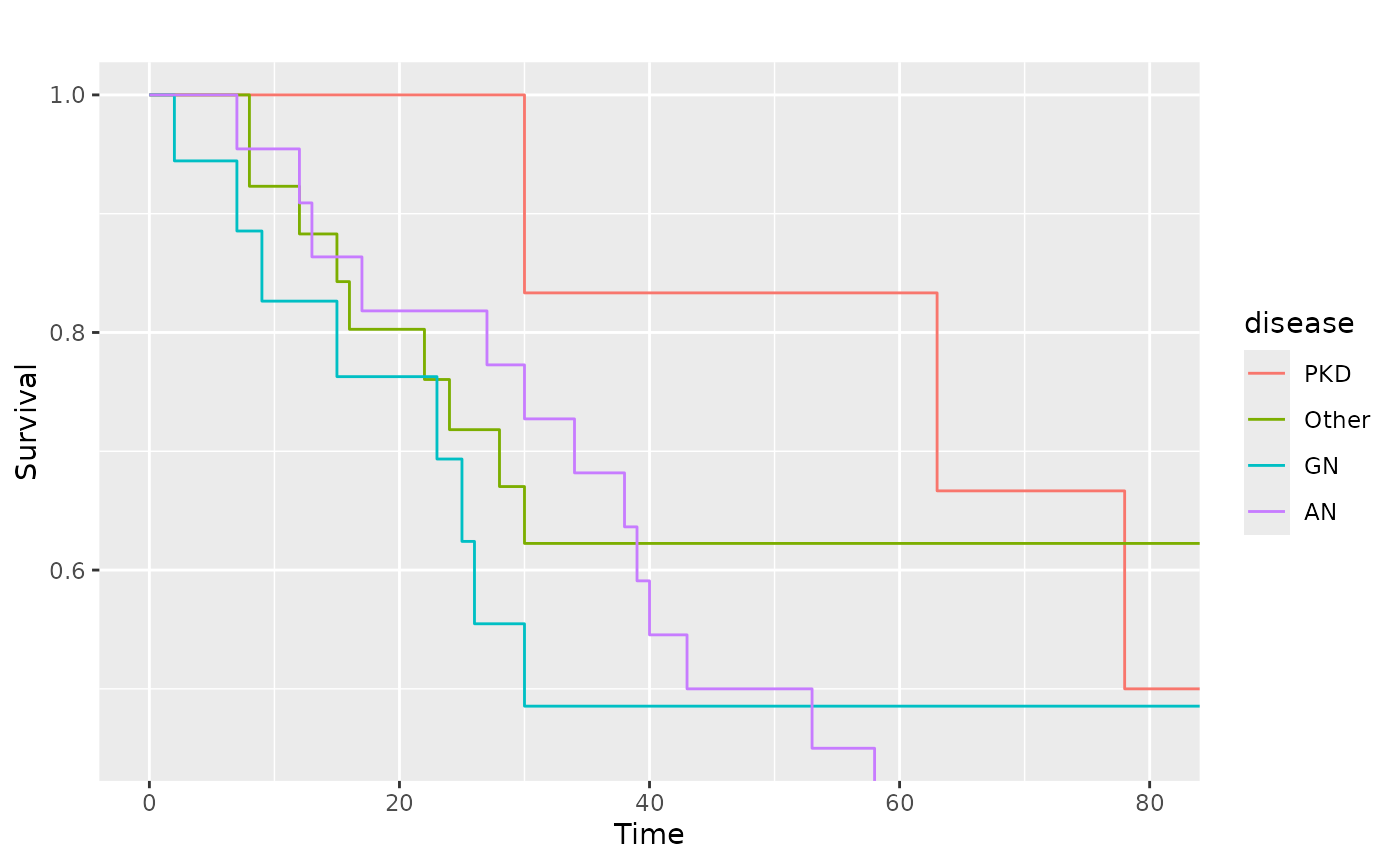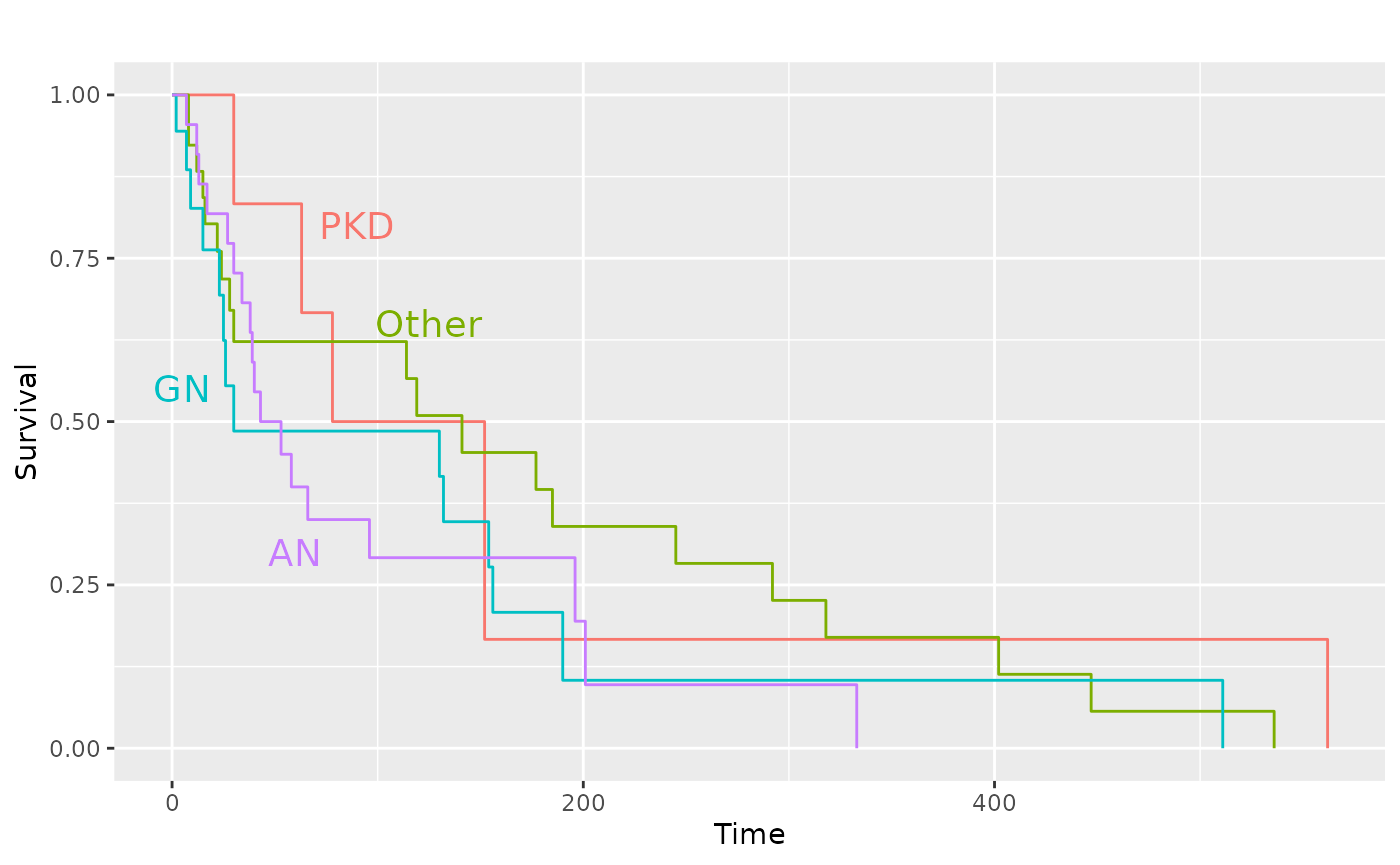library(GGally)
#> Registered S3 method overwritten by 'GGally':
#>   method from
#>   +.gg   ggplot2

## GGally::ggsurv()

This function produces Kaplan-Meier plots using ggplot2. As a first argument, ggsurv() needs a survival::survfit() object. Default settings differ for single stratum and multiple strata objects.

### Single Stratum

require(ggplot2)
require(survival)
require(scales)

data(lung, package = "survival")
#> Warning in data(lung, package = "survival"): data set 'lung' not found
sf.lung <- survival::survfit(Surv(time, status) ~ 1, data = lung)
ggsurv(sf.lung)### Multiple Stratum

The legend color positions matches the survival order or each stratum, where the stratums that end at a lower value or time have a position that is lower in the legend.

sf.sex <- survival::survfit(Surv(time, status) ~ sex, data = lung)
pl.sex <- ggsurv(sf.sex)
pl.sex### Alterations

Since a ggplot2 object is returned, plot objects may be altered after the original creation.

pl.sex +
ggplot2::guides(linetype = "none") +
ggplot2::scale_colour_discrete(
name   = "Sex",
breaks = c(1, 2),
labels = c("Male", "Female")
)
#> Scale for colour is already present.
#> Adding another scale for colour, which will replace the existing scale.data(kidney, package = "survival")
#> Warning in data(kidney, package = "survival"): data set 'kidney' not found
sf.kid <- survival::survfit(Surv(time, status) ~ disease, data = kidney)
pl.kid <- ggsurv(sf.kid, plot.cens = FALSE)
pl.kid# Zoom in to first 80 days
pl.kid + ggplot2::coord_cartesian(xlim = c(0, 80), ylim = c(0.45, 1))#### Add text and remove the legend

pl.kid +
ggplot2::annotate(
"text",
label = c("PKD", "Other", "GN", "AN"),
x = c(90, 125, 5, 60),
y = c(0.8, 0.65, 0.55, 0.30),
size = 5,
colour = scales::hue_pal(
h         = c(0, 360) + 15,
c         = 100,
l         = 65,
h.start   = 0,
direction = 1
)(4)
) +
ggplot2::guides(color = "none", linetype = "none")